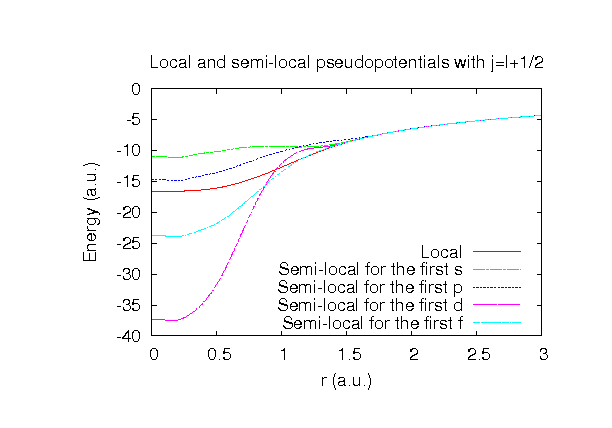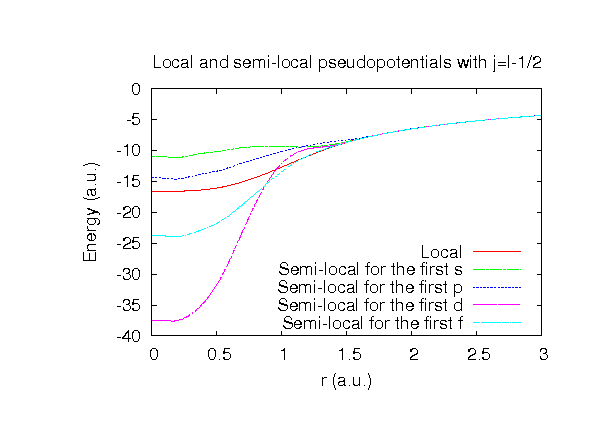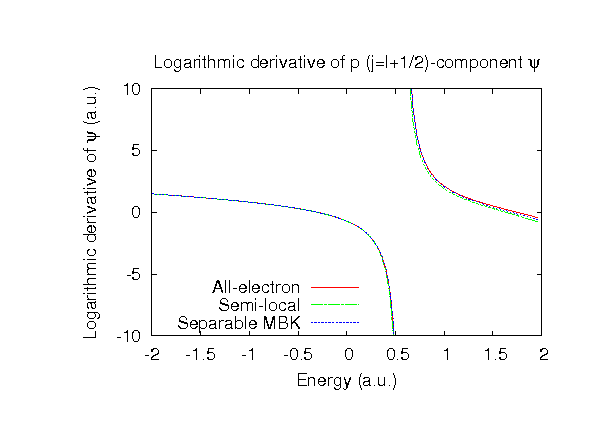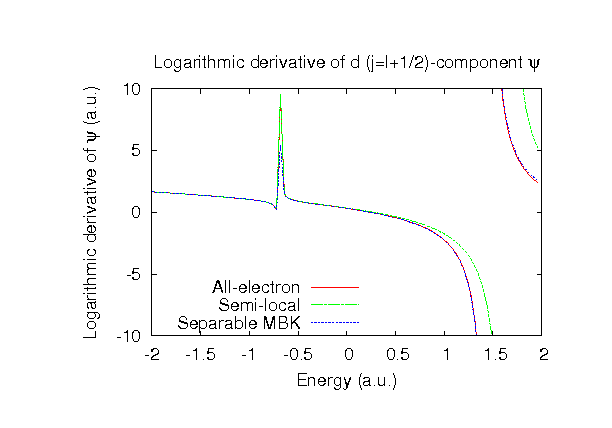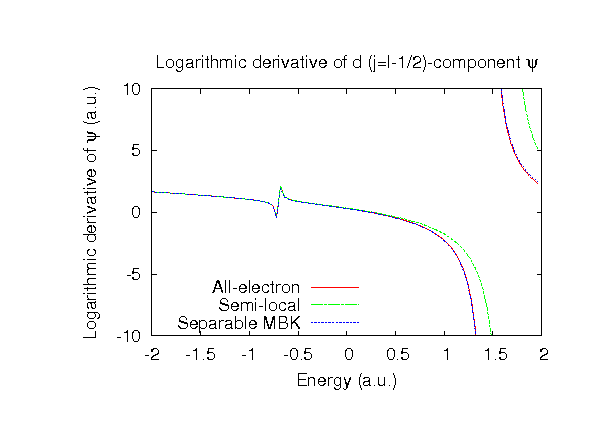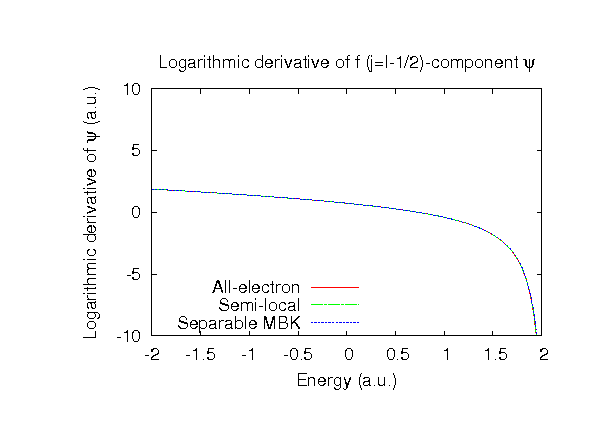### Fully relativistic pseudopotentials

Fully relativistic pseudopotentials generated by the MBK (PRB 47, 6728 (1993)) scheme within LDA (CA13) and GGA (PBE13) which contain a partial core correction and fully relativistic effects including spin-orbit coupling.

### Pseudo-atomic orbitals

The number below the symbol means a cutoff radius (a.u.) of the confinement potential. These file includes fifteen radial parts for each angular momentum quantum number l (=0,1,2,3,4). The basis functions were generated by variationally optimizing the corresponding primitive basis functions in the single atom and the BCC bulk. The input files used for the orbital optimization can be found at Ga_opt.dat and Gabcc_opt.dat . Since Ga_CA13.vps and Ga_PBE13.vps include the 3d, 4s, and 4p states (13 electrons) as the valence states, the minimal basis set is Ga*.*-s1p1d1. Our recommendation for the choice of cutoff radius of basis functions is that Ga7.0.pao is enough for bulks, but Ga8.0.pao or Ga9.0.pao is preferable for molecular systems.

### Benchmark calculations by the PBE13 pseudopotential with the various basis functions

(1) Calculation of the total energy as a function of lattice constant in the bcc structure, where the total energy is plotted relative to the minimum energy for each case. a0 and B0 are the equilibrium lattice constant and bulk modulus obtained by fitting to the Murnaghan equation of state. The difference between Ga7.0-s2p2d2 and Ga7.0-s3p3d3f1 in the total energy at the minimum point is 0.0377 eV/atom. An input file used for the OpenMX calculations can be found at Gabcc-EvsV.dat . For comparison the result by the Wien2k code is also shown, where the calculation was performed by default setting in the Ver. 10.1 of Wien2k except for the use of RMT x KMAX of 12.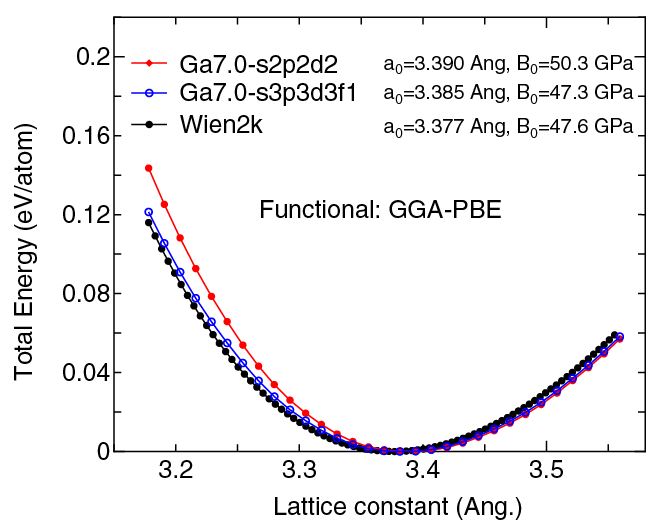(2) Calculations of the band dispersion in the bcc structure, where the non-spin polarized collinear calculation with the lattice constant of 3.378 Ang. was performed using Ga_PBE13.vps and Ga8.0-s2p2d2 and the origin of the energy is taken to be the Fermi level. The input file used for the OpenMX calculations can be found at Gabcc-Band.dat . For comparison the result by the Wien2k code is also shown, where the calculation was performed by default setting in the Ver. 10.1 of Wien2k except for the use of RMT x KMAX of 12.(3) Calculations of the Ga2 molecule with S=3, where Ga_PBE13.vps and Ga9.0-s2p2d2 or Ga9.0-s3p3d3f1 were used. The input files used for the OpenMX calculations can be found at Ga2.dat , Ga.dat , and Ga_CP.dat .

 Equilibrium bond length (Ang.) Atomization energy (eV) Atomization energy (couterpoise corrected) (eV) Ga9.0-s2p2d2 2.823 1.583 1.567 Ga9.0-s3p3d3f1 2.785 1.683 1.661 Other calc. 2.795 a 1.46 a Expt. 1.102 b

a BLYP result: Y. Zhao et al., J. Phys. Chem. A 108, 7448 (2004).
b X. Tan and P.J. Dagdigian, J. Phys. Chem. A 107, 2642 (2003).

### Supplementary information for the GGA (PBE13) pseudopotential